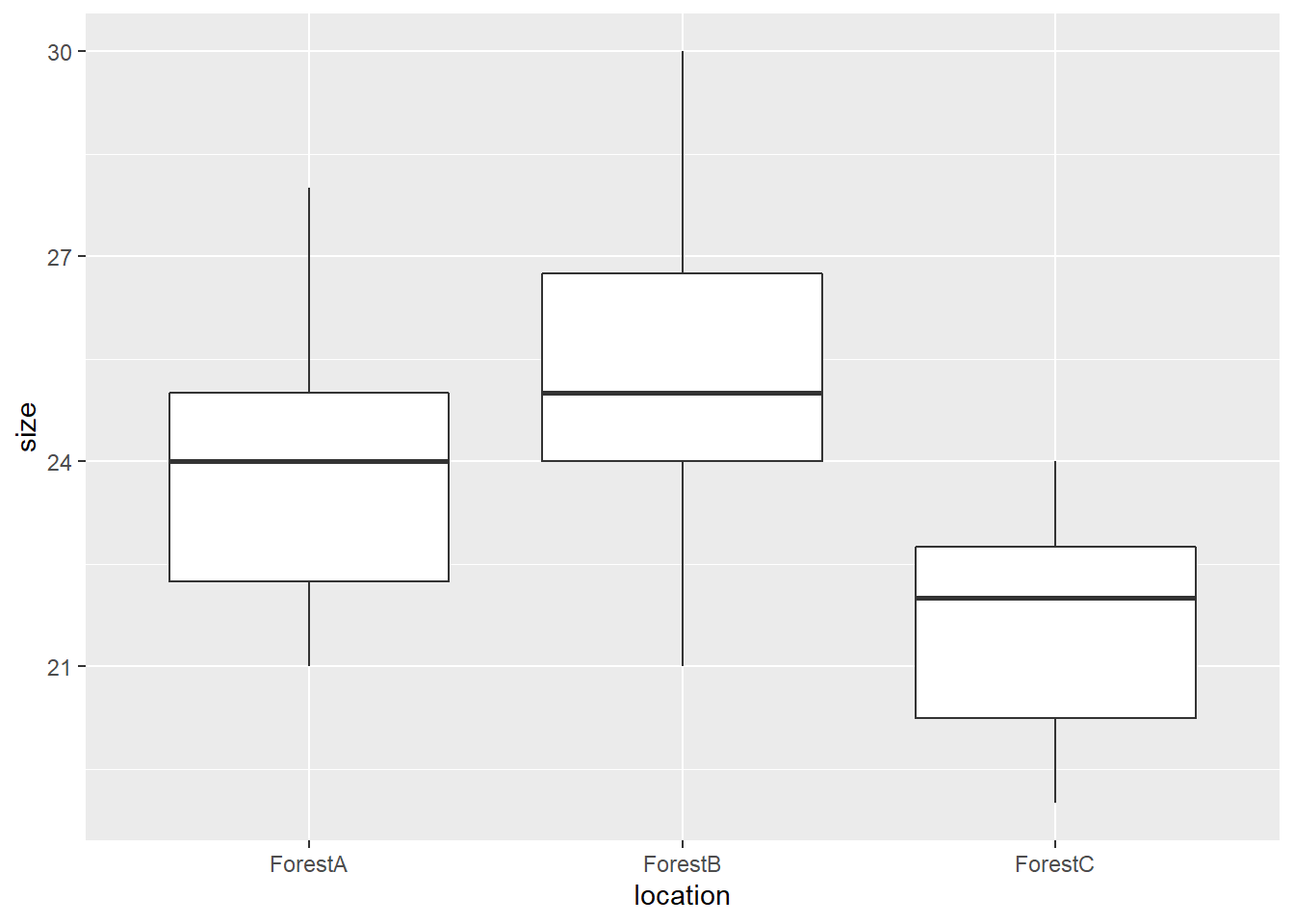2 tests are commonly used to check for homogeneity of variance: Fisher’s F test and Levene’s test. Fisher’s F test, which is introduced here, is restricted to comparison of two variances/groups while Levene’s test can assess more than two variances/groups. Like normality, verifying homogeneity of variance is a requirement for running several of the well-known statistical tests such as Student’s t-test and ANOVA.

Here we will see how to perform Levene’s test. In this test, the null hypothesis H0 is that all variances are equal. Note that this test is meant to be used with normally distributed data, but can tolerate relatively low deviation from normality. The corresponding function in R is leveneTest() and the syntax is leveneTest(response ~ predictor, data, center) where response is the response variable, predictor is the predictor variable that contains the groups to compare and data is the dataframe. One can also add the optional argument center = mean or center = median to define how the function will compute the center of each group. mean gives the original Levene’s test; the default, median, provides a more robust test called Brown-Forsythe test for homogeneity of variance.

leveneTest() is part of the package car, so start with activating it:

library(car)

Here we will consider the same example as introduced here:

# response variable
size <- c(25,22,28,24,26,24,22,21,23,25,26,30,25,24,21,27,28,23,25,24,20,22,24,23,22,24,20,19,21,22)

# predictor variable
location <- c(rep("ForestA",10), rep("ForestB",10), rep("ForestC",10))

# dataframe
my.dataframe <- data.frame(size,location)

We may first visualize the data with a boxplot:Running the test

The syntax adapted to our example is as follow:

leveneTest(size ~ location, my.dataframe, center=mean)
## Levene's Test for Homogeneity of Variance (center = mean)
##       Df F value Pr(>F)
## group  2  0.5636 0.5757
##       27

The test reveals a p-value greater than 0.05, indicating that there is no significant difference between the group variances in location.

Alternative

The Fligner-Killeen test does a rather similar job, meaning that it checks for homogeneity of variance, but is a better option when data are non-normally distributed or when problems related to outliers in the dataset cannot be resolved.

The function is fligner.test() and the syntax is fligner.test(response ~ predictor, data) which is very similar leveneTest( ).

fligner.test(size ~ location, my.dataframe)
##
##  Fligner-Killeen test of homogeneity of variances
##
## data:  size by location
## Fligner-Killeen:med chi-squared = 0.9556, df = 2, p-value = 0.6201

and again the p-value is greater than 0.05; the variances are homogeneous.